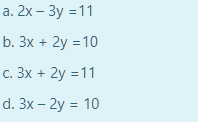select one

reopened

3y=2x-9 (rewriting the given equation), which has a slope ⅔. The perpendicular slope is -1/⅔=-3/2.

So we are looking for this gradient in the given answer options. This means y=-3x/2+a where a is a constant. That is, 2y=-3x+2a, or 3x+2y=2a. There are actually two possible answers b or c, because 2a can be even or odd. I suspect that the expected answer is option b.

by Top Rated User (1.1m points)
selected by